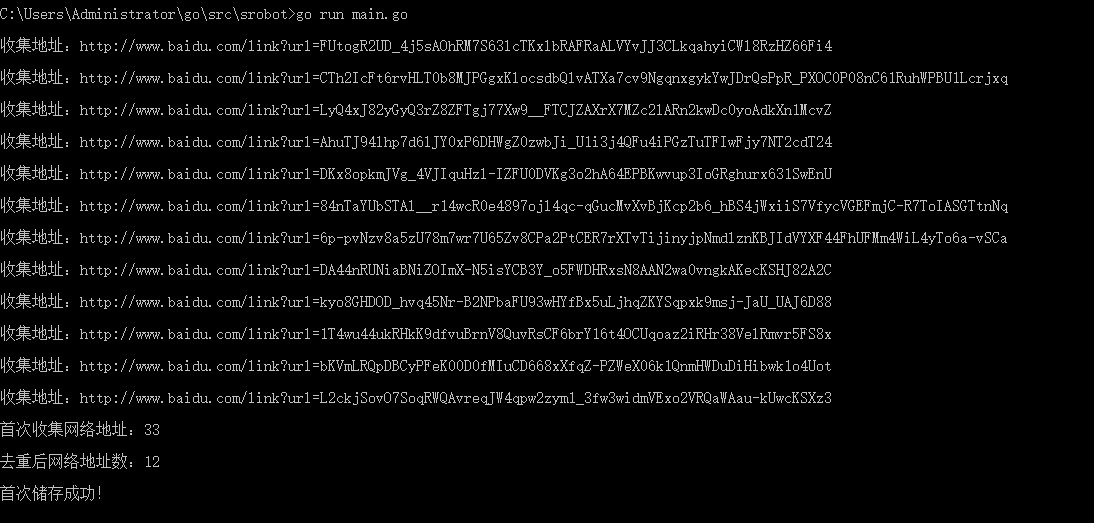# Golang原生爬虫 简单爬虫实现 不依赖第三方包库 方便理解技术原理 （一）

Golang原生爬虫 简单爬虫实现 不依赖第三方包库 方便理解技术原理 （二）

import (
"fmt"
"io"
"io/ioutil"
"net/http"
"os"
"regexp"
"strconv"
"strings"
"time"
)var (
regHref       = ((ht|f)tps?)://[w]{0,3}.baidu.com/link\?[a-zA-z=0-9-\s]*
)

func Timer() {
t := time.NewTimer(time.Second * 1)
<-t.C
fmt.Print("\n\n\n执行爬抓\n\n")
Timer()
}

func main() {
if checkFile("./data/", "url.txt").Size() == 0 {
fistStart()
main()
} else {
Timer()
}
}

func fistStart() {
var num int
url := "http://www.baidu.com/s?ie=utf-8&f=8&rsv_bp=1&tn=39042058_20_oem_dg&wd=golang%E5%AE%9E%E7%8E%B0&oq=golang%2520%25E5%2588%25A0%25E9%2599%25A4%25E6%2595%25B0%25E7%25BB%2584&rsv_pq=d9be28ec0002df1b&rsv_t=8017GWpSLPhDmKilZQ1StC04EVpUAeLEP90NIm%2Bk5pRh5R9o57NHMO8Gaxm1TtSOo%2FvtJj%2B98%2Fsc&rqlang=cn&rsv_enter=1&inputT=3474&rsv_sug3=16&rsv_sug1=11&rsv_sug7=100&rsv_sug2=0&rsv_sug4=4230"
resp, _ := http.Get(url)
defer resp.Body.Close()
body, _ := ioutil.ReadAll(resp.Body)
reg := regexp.MustCompile(((ht|f)tps?)://[w]{0,3}.baidu.com/link\?[a-zA-z=0-9-\s]*)
f, _ := os.OpenFile("./data/url.txt", os.O_CREATE|os.O_APPEND|os.O_RDWR, 0666)
defer f.Close()
for _, d := range reg.FindAllString(string(body), -1) {
ff, _ := os.OpenFile("./data/url.txt", os.O_RDWR, 0666)
file, _ := ioutil.ReadAll(ff)
dd := strings.Split(d, "")
dddd := ""
for _, ddd := range dd {
if ddd == "?" {
ddd = \?
}
dddd += ddd
}
if checkRegexp(string(file), dddd, 0).(string) == "" {
io.WriteString(f, d+"\n")
fmt.Print("\n收集地址：" + d + "\n")
num++
}
// fmt.Print(string(file))
ff.Close()
}
fmt.Print("\n首次收集网络地址：" + strconv.Itoa(len(reg.FindAllString(string(body), -1))) + "\n")
fmt.Print("\n去重后网络地址数：" + strconv.Itoa(num))
fmt.Print("\n\n首次储存成功！\n")
}

	url := "http://www.baidu.com/s?ie=utf-8&f=8&rsv_bp=1&tn=39042058_20_oem_dg&wd=golang%E5%AE%9E%E7%8E%B0&oq=golang%2520%25E5%2588%25A0%25E9%2599%25A4%25E6%2595%25B0%25E7%25BB%2584&rsv_pq=d9be28ec0002df1b&rsv_t=8017GWpSLPhDmKilZQ1StC04EVpUAeLEP90NIm%2Bk5pRh5R9o57NHMO8Gaxm1TtSOo%2FvtJj%2B98%2Fsc&rqlang=cn&rsv_enter=1&inputT=3474&rsv_sug3=16&rsv_sug1=11&rsv_sug7=100&rsv_sug2=0&rsv_sug4=4230"
resp, _ := http.Get(url)
defer resp.Body.Close()
body, _ := ioutil.ReadAll(resp.Body)
reg := regexp.MustCompile(((ht|f)tps?)://[w]{0,3}.baidu.com/link\?[a-zA-z=0-9-\s]*)
f, _ := os.OpenFile("./data/url.txt", os.O_CREATE|os.O_APPEND|os.O_RDWR, 0666)
defer f.Close()

	for _, d := range reg.FindAllString(string(body), -1) {
ff, _ := os.OpenFile("./data/url.txt", os.O_RDWR, 0666)
file, _ := ioutil.ReadAll(ff)
dd := strings.Split(d, "")
dddd := ""
for _, ddd := range dd {
if ddd == "?" {
ddd = \?
}
dddd += ddd
}
if checkRegexp(string(file), dddd, 0).(string) == "" {
io.WriteString(f, d+"\n")
fmt.Print("\n收集地址：" + d + "\n")
num++
}
// fmt.Print(string(file))
ff.Close()
}

checkRegexp函数：

func checkRegexp(cont string, reg string, style int) (result interface{}) {
check := regexp.MustCompile(reg)
switch style {
case 0:
result = check.FindString(cont)
case 1:
result = check.FindAllString(cont, -1)
default:
result = check.FindAll([]byte(cont), -1)
}
return
}Golang原生爬虫 简单爬虫实现 不依赖第三方包库 方便理解技术原理 （二）